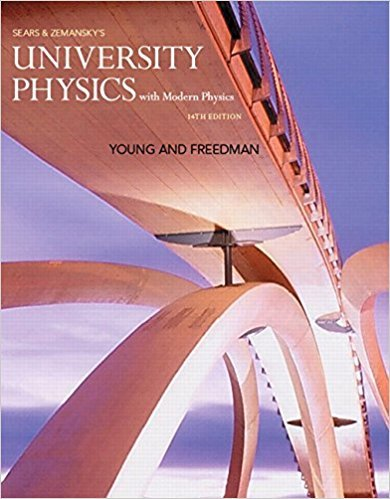×
×

# Answer: At launch a rocket ship weighs 4.5 million pounds.ISBN: 9780321973610 228

## Solution for problem 2.29 Chapter 2

University Physics with Modern Physics (1) | 14th Edition

• Textbook Solutions
• 2901 Step-by-step solutions solved by professors and subject experts
• Get 24/7 help from StudySoup virtual teaching assistantsUniversity Physics with Modern Physics (1) | 14th Edition

4 5 1 303 Reviews
13
1
Problem 2.29

At launch a rocket ship weighs 4.5 million pounds. When it is launched from rest, it takes 8.00 s to reach 161 km>h; at the end of the first 1.00 min, its speed is 1610 km>h. (a) What is the average acceleration (in m>s 2 ) of the rocket (i) during the first 8.00 s and (ii) between 8.00 s and the end of the first 1.00 min? (b) Assuming the acceleration is constant during each time interval (but not necessarily the same in both intervals), what distance does the rocket travel (i) during the first 8.00 s and (ii) during the interval from 8.00 s to 1.00 min?

Step-by-Step Solution:
Step 1 of 3

Reading Graphs:  Impulse J = Force x change in time  p = mv  Only next impulse equals change in momentum  These quantities are vectors  The impulse of the graph can be determined by: the area under a Force vs Time F(t) graph  Why It’s Important o Force plates are commonly used to study a variety of things  Biomechanics,...

Step 2 of 3

Step 3 of 3

##### ISBN: 9780321973610

Unlock Textbook Solution